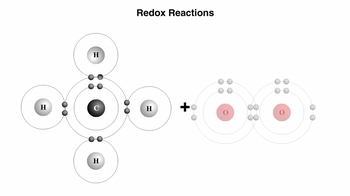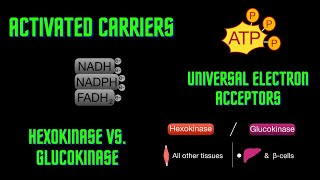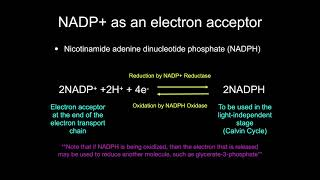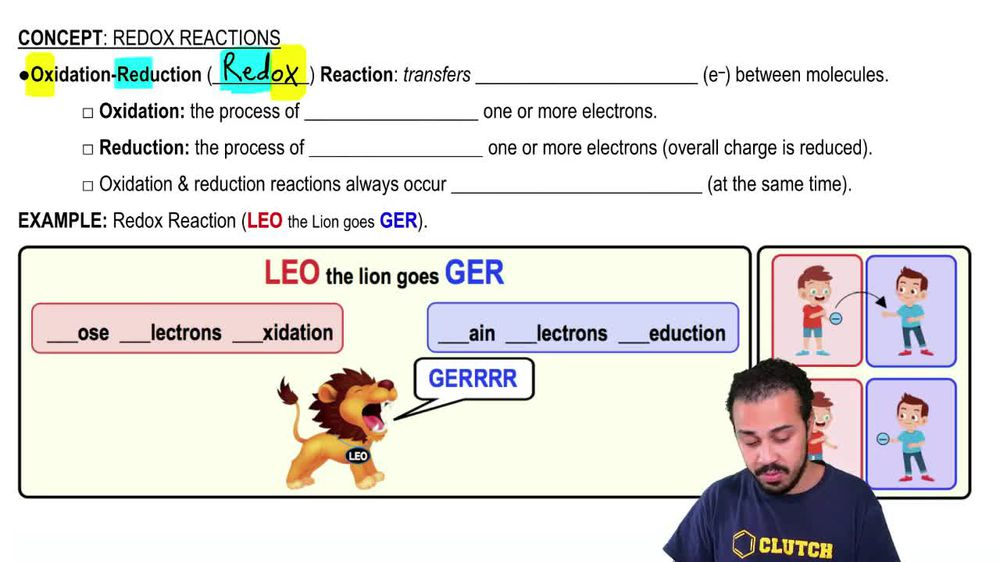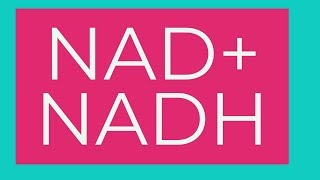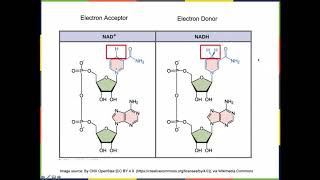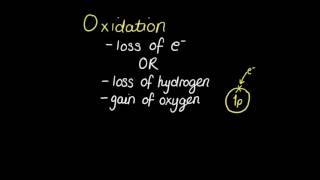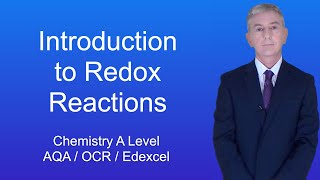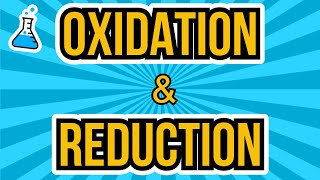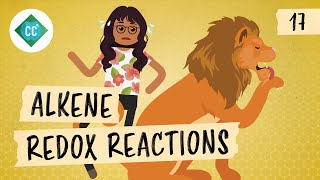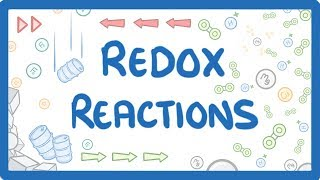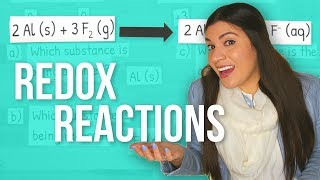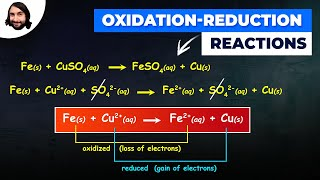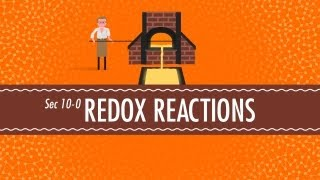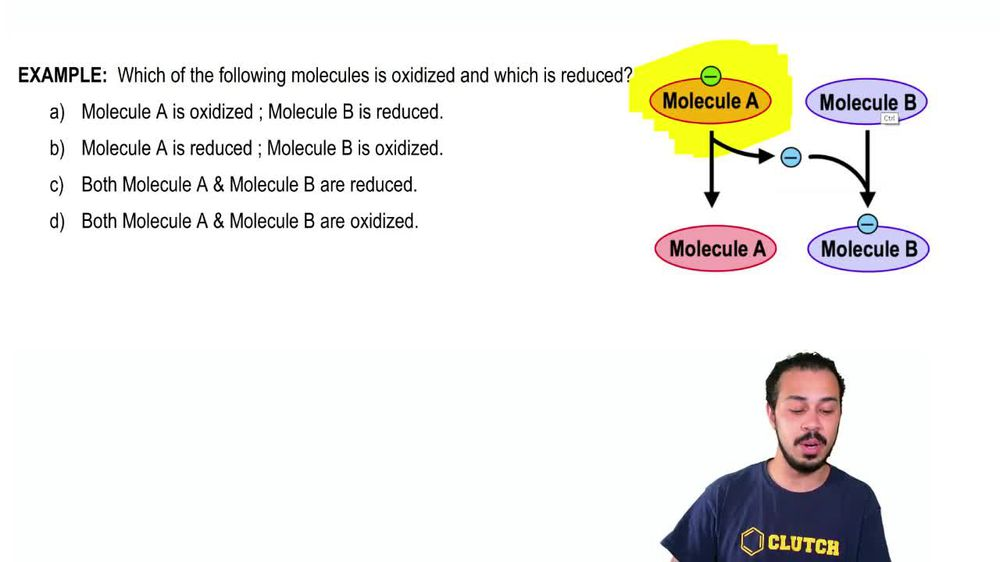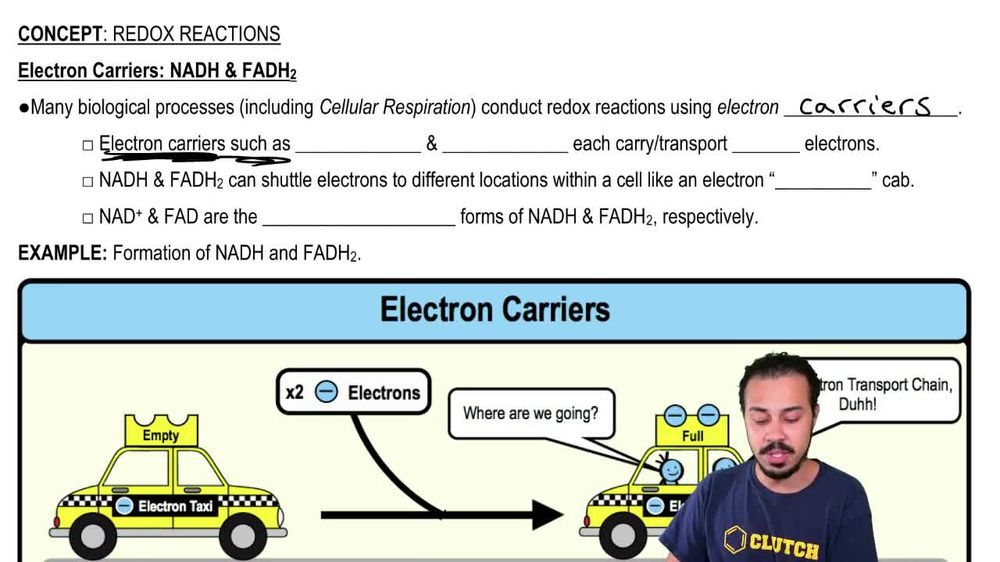Start typing, then use the up and down arrows to select an option from the list.
1. 8. Respiration2. Redox Reactions# Redox Reactions Example 1

by Jason Amores Sumpter
335 views
4
4
So here we have an example problem that's asking which of the following molecules is oxidized and which is reduced and notice that we have molecule a over here and molecule B and they are undergoing a chemical reaction. And so notice we have these four potential answer options over here. And so, of course, what we need to recall from our last lesson video is that when it comes toe oxidation reduction reactions or redox reactions, really, all we need to remember is Leo the lion goes ger and because if we remember Leo the lion goes ger, then we'll be able to remember that oxidation is when a substance loses electrons and reduction is when a substance gains electrons. And so, looking at this reaction here between molecule a molecule be noticed that molecule a is starting with an electron here, and blue and molecule B seems to not have those that electron and so notice that after this reaction, molecule A is actually donating its electron two molecule B, and so molecule A is losing an electron by the end of the reaction, whereas molecule B is gaining the electron. And so because molecule B is gaining the electron that makes it reduced. And because molecule A is losing the electron by the end of the reaction that makes molecule a oxidized. And so we need to choose the option that corresponds with that and notice option A says molecule is oxidized, whereas molecule B is reduced. And that is going to be the correct answer to this example. Problems. So we could go ahead and mark a here as correct. And that concludes this example problems, so I'll see you all in our next video.